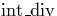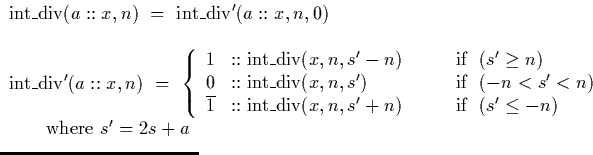Next: (Mantissa, Exponent) Operations Up: Signed Binary Stream Operations Previous: Multiplication

## Division by an integer

A third primitive operation on streams of signed binary digits is division of such a stream by an integer. Although it is not in general possible to divide a stream representing a number in the interval [-1,1] by another stream representing a number in the interval [-1,1] (as this interval is not closed under division), division by an integer value may be performed quite simply. An algorithm for division of arbitrary numbers in the signed binary (mantissa, exponent) representation is described in section 4.4.4.

The method used is similar to the school long division method, although both the input and output streams may contain negative digits. Digits are examined at the head of the numerator stream and evaluated as if they formed a signed binary integer. If this sum exceeds the denominator a one is output and the sum decremented by the denominator. Similarly if this sum becomes less than the negated denominator a minus one is output and the sum incremented by the denominator. If the absolute value of the sum is smaller than the denominator, we generate a zero digit.

A functionwhich divides a stream of the form (a::x) by the integer n may be defined as follows:Next: (Mantissa, Exponent) Operations Up: Signed Binary Stream Operations Previous: Multiplication
Martin Escardo
5/11/2000